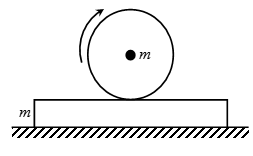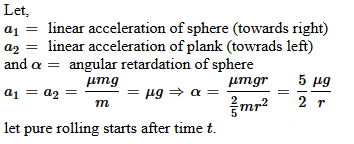×#### Thank you for registering.

One of our academic counsellors will contact you within 1 working day.

Click to Chat

1800-1023-196

+91-120-4616500

CART 0

• 0

MY CART (5)

Use Coupon: CART20 and get 20% off on all online Study Material

ITEM
DETAILS
MRP
DISCOUNT
FINAL PRICE
Total Price: Rs.

There are no items in this cart.
Continue Shopping
```
A solid sphere of mass m and radius R is placed on a plank of equal mass, which lies in a smoth horizontal surface.The sphere is given a sharp impulse as a result it stats slidding with velocity v.Find the time taken by the sphere to start pure roliing on the plank.the coefficient of friction between plank and sphere is k.

```
9 years ago

```							velocity of center of mass is v & angular velocity of sphere is 0 initially...
friction will act in backward direction ....now
f = kmg                           (f = friction force)
ma = kmg
a=-kmg       (a is retardation of center of mass)
now , torque = fR = I(alfa)                        (alfa = angular accleration)
alfa = fR/I
= 5kg/2R                              (Isphere = 2MR2/5)
now after time t let velocity of ceneter of mass  is V then
V = U + at                             (initial linear velocity is v)
V = v - kgt         ............1
let at this time angular velocity os W then
W = Wo + (alfa)t                                (initial angular velocity is 0)
W = 5kgt/2R                 ............2
now if pure rolling has started then V = WR
so , v - kgt = 5kgt/2
t = 2v/7kg
```
9 years ago
```							Initially velocity of plank is 0 and of disc is v.  friction will act in backward direction ....nowf = kmg                           (f = friction force) ma = kmga = - kg            (a is retardation of center of mass)now , torque = fR = I(alfa)                (alfa = angular accleration)alfa = fR/I alfa = 5gk/2R                        (I sphere = 2MR2/5)On plank friction will act on forward direction hence acceleration is in forward direction. f = makmg = ma `a` of plank is kg Let velocity of plank at time t is V1 v = u + atV1 = kgtSimilarly velocity of disc at time t is V2 = v - kgtAnd W (angular velocity) at time t is W = 2kgt/rSo velocity of contact point is  = (v - kgt) - (2kgt/r)×r = v - 3kgtFor pure rolling kgt = v - 3kgt4kgt = vt = v/4kg

The above answer become so conjusted so I am sending this again.                                                                 f = kmg (f =  friction force).                                             ma = kmg.                a = - kg.           (a is retardation of center of mass).    torque = fR = I(alfa).   (alfa = angular acceleration)Alfa = fR/I                          alfa = 5gk/2R              (I sphere = 2MR2/5).                                                                  On plank  friction will act on forward direction hence acceleration is in forward  direction.                                                f = ma.             kmg = ma.                       a = kg                                     Let velocity of plank at time t is V1.          v = u + at.                        V1 = kgt.                             Similarly  velocity of disc at time t is              V2 = v - kgt.                      And W (angular velocity) at time t is.                W = 2kgt/r.                     So velocity of contact point is = (v - kgt) - (2kgt) =  v - 3kgt.                For pure rolling                                                                         kgt = v - 3kgt.                              4kgt = v.                                     t = v/4kg
```
4 years ago605 Points
```							Dear student,Please find the attached solution to your problem below.Here, u = k (coefficient of friction)Now, during pure rollingVs – wr = Vpv – kgt – 5kgt/2r * r = kgtor, v = 9/2 kgtHence, t = 2v / 9kg Hope it helps.Thanks and regards,Kushagra
```
3 months ago
Think You Can Provide A Better Answer ?

## Other Related Questions on Mechanics

View all Questions »### Course Features

• 101 Video Lectures
• Revision Notes
• Previous Year Papers
• Mind Map
• Study Planner
• NCERT Solutions
• Discussion Forum
• Test paper with Video Solution### Course Features

• 110 Video Lectures
• Revision Notes
• Test paper with Video Solution
• Mind Map
• Study Planner
• NCERT Solutions
• Discussion Forum
• Previous Year Exam Questions